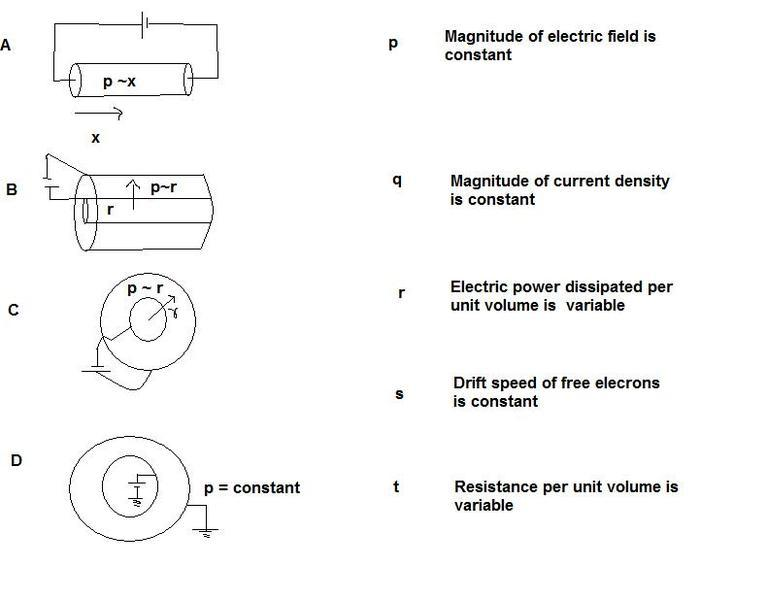# Current Electricity

## Homework Statement

Refer figureNote: ~ stands for proportionality sign and p stands for resistivity.

## The Attempt at a Solution

R=pl/A
Rnet~l2/A
V is constant across each conductor, so magnitude of electric field must be constant for every case. Since drift velocity depends on electric field, it is also constant for all. Wrong

Total current I in the circuit is constant in every case. But current density is constant only in A as area perpendicular to the current flow is constant. Correct.

Resistance per unit volume and Power dissipated per unit volume both are variables for all as both are functions of distance. Correct.

The only problem is with drift velocity and electric field.

SammyS
Staff Emeritus
Homework Helper
Gold Member

## Homework Statement

Refer figure

https://www.physicsforums.com/attachment.php?attachmentid=32966&stc=1&d=1299855441"

Note: ~ stands for proportionality sign and p stands for resistivity.

## The Attempt at a Solution

R=pl/A
Rnet~l2/A
V is constant across each conductor, so magnitude of electric field must be constant for every case. Since drift velocity depends on electric field, it is also constant for all. Wrong

Total current I in the circuit is constant in every case. But current density is constant only in A as area perpendicular to the current flow is constant. Correct.

Resistance per unit volume and Power dissipated per unit volume both are variables for all as both are functions of distance. Correct.

The only problem is with drift velocity and electric field.

It's not too clear to me what the objects in C and D look like in three dimensions. Also, the configuration of the electrodes in C is not clear.

Use the differential form of your equation:

$$dR=\frac{\rho}{A}\,d\ell\,,$$

then integrate.

Also, $$I=\int_S\vec{J}\cdot\vec{dA}\,,$$

$$dV=-\vec{E}\cdot\vec{d\ell}$$

and $$\vec{E}=\rho\vec{J}\,,$$

Last edited by a moderator:
I still don't get the answer.
Using your expressions, I got that E depends on x or r in every case i.e. it is not constant.
option (p) is included for (B) and (C) and option (s) for (A) only.

SammyS
Staff Emeritus
Homework Helper
Gold Member
It's still not clear to me: what objects are in C & D !

Spheres with spherical cavities? (Then how are they different? Is part of the electrode buried in C?)

Looking at the end of cylinders?

Ellipsoid with spherical cavity, or sphere with elliptical cavity.

Why is part of the wire going to the inside of C straight?

This question now seems unclear to me too. Lets close this topic.